﻿ 数据结构——二叉树的顺序结构---堆

### 数据结构——二叉树的顺序结构---堆

2021-11-26 9:17:43

### 文章目录

• 前言
• 一、二叉树的顺序结构
• 二、堆的概念及结构
• 三、堆的实现
• 1.向下调整算法
• 2.堆的创建
• 四、堆的整体代码
• 五、建堆的时间复杂度
• 六、堆排序以及top-k问题
• 1.堆排序
• 2.TOP-K问题
• 总结

# 一、二叉树的顺序结构# 二、堆的概念及结构

• 堆中某个节点的值总是不大于或不小于其父节点的值；
• 堆总是一棵完全二叉树。# 三、堆的实现

## 1.向下调整算法``````//向下调整
void AdjustDown(HPDataType* a, int n, int parent)
{
int child = parent * 2 + 1;//默认左孩子小于右孩子
while (child < n)
{
if (child + 1 < n && a[child + 1] < a[child])//右孩子小于左孩子就换一下
{
child++;
}
if (a[child] < a[parent])
{
swap(&a[parent], &a[child]);
parent = child;
child = parent * 2 + 1;
}
else
{
break;
}
}
}
``````## 2.堆的创建``````void heap(int* a, int n)
{
for (int i = (n - 2) / 2; i >= 0; i--)
{
}
}
``````# 四、堆的整体代码

``````//打印堆中的数据
void HeapPrint(Heap* hp)
{
for (int i = 0; i < hp->size; i++)
{
printf("%d ", hp->a[i]);
}
printf("\n");
}
//交换堆中的两个数据
void swap(HPDataType* a, HPDataType* b)
{
HPDataType tmp = *a;
*a = *b;
*b = tmp;
}

//向上调整
{
assert(a);
int parent = (child - 1) / 2;
while (child > 0)
{
if (a[child] < a[parent])
{
swap(&a[child], &a[parent]);
child = parent;
parent = (child - 1) / 2;
}
else
{
break;
}
}
}

//向下调整
void AdjustDown(HPDataType* a, int n, int parent)
{
int child = parent * 2 + 1;//默认左孩子小于右孩子
while (child < n)
{
if (child + 1 < n && a[child + 1] < a[child])//右孩子小于左孩子就换一下
{
child++;
}
if (a[child] < a[parent])
{
swap(&a[parent], &a[child]);
parent = child;
child = parent * 2 + 1;
}
else
{
break;
}
}
}
// 堆的构建
void HeapInit(Heap* hp)
{
assert(hp);
hp->a = NULL;
hp->capacity = hp->size = 0;
}
// 堆的销毁
void HeapDestory(Heap* hp)
{
assert(hp);
free(hp->a);
hp->a = NULL;
hp->capacity = hp->size = 0;
}
// 堆的插入
void HeapPush(Heap* hp, HPDataType x)
{
assert(hp);
if (hp->capacity == hp->size)
{
int newcapacity = hp->capacity == 0 ? 4 : hp->capacity * 2;
HPDataType* tmp = (HPDataType*)realloc(hp->a, sizeof(HPDataType)*newcapacity);
if (tmp == NULL)
{
printf("realloc fail\n");
}
hp->a = tmp;
hp->capacity = newcapacity;
}
hp->a[hp->size] = x;
hp->size++;

}
// 堆顶数据的删除
void HeapPop(Heap* hp)
{
assert(hp);
assert(!HeapEmpty(hp));
swap(&hp->a, &hp->a[hp->size - 1]);
hp->size--;
}
// 取堆顶的数据
HPDataType HeapTop(Heap* hp)
{
assert(hp);
assert(!HeapEmpty(hp));
return hp->a;
}
// 堆的数据个数
int HeapSize(Heap* hp)
{
assert(hp);
return hp->size;
}
// 堆的判空
bool HeapEmpty(Heap* hp)
{
assert(hp);
return hp->size == 0;
}
``````# 五、建堆的时间复杂度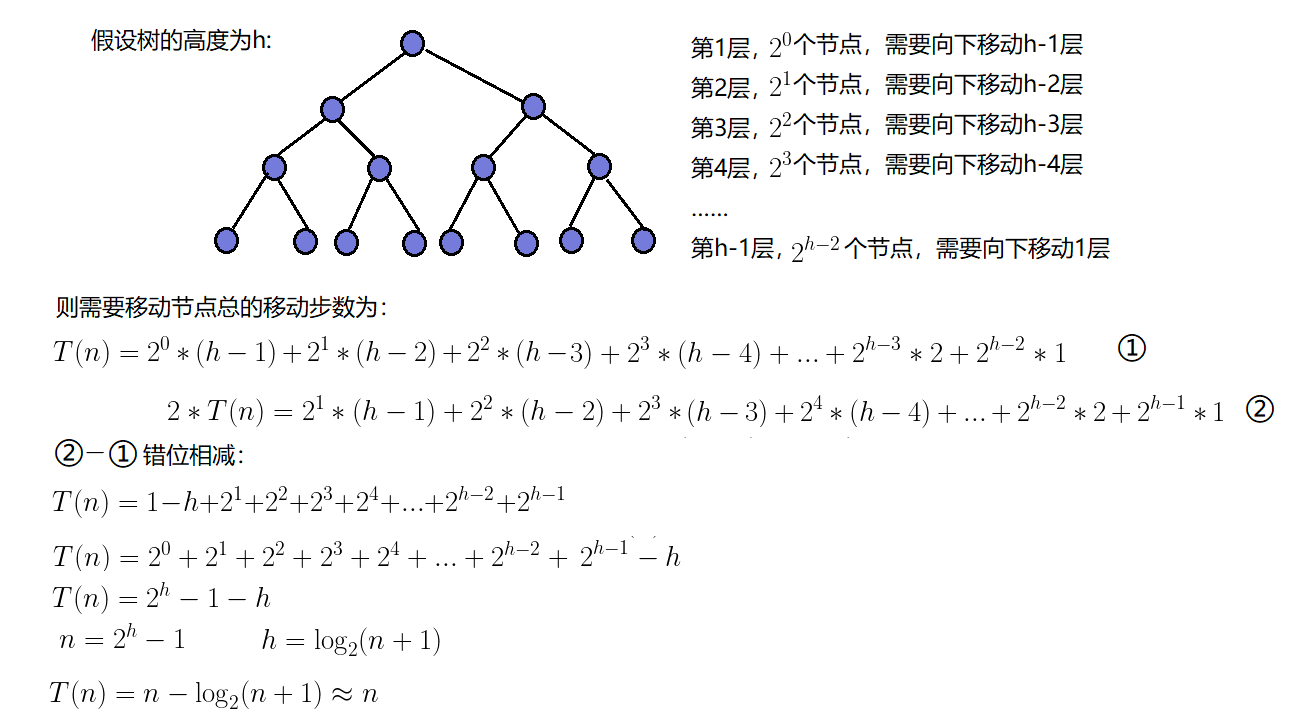# 六、堆排序以及top-k问题

## 1.堆排序

1.建堆

• 升序：建大堆
• 降序：建小堆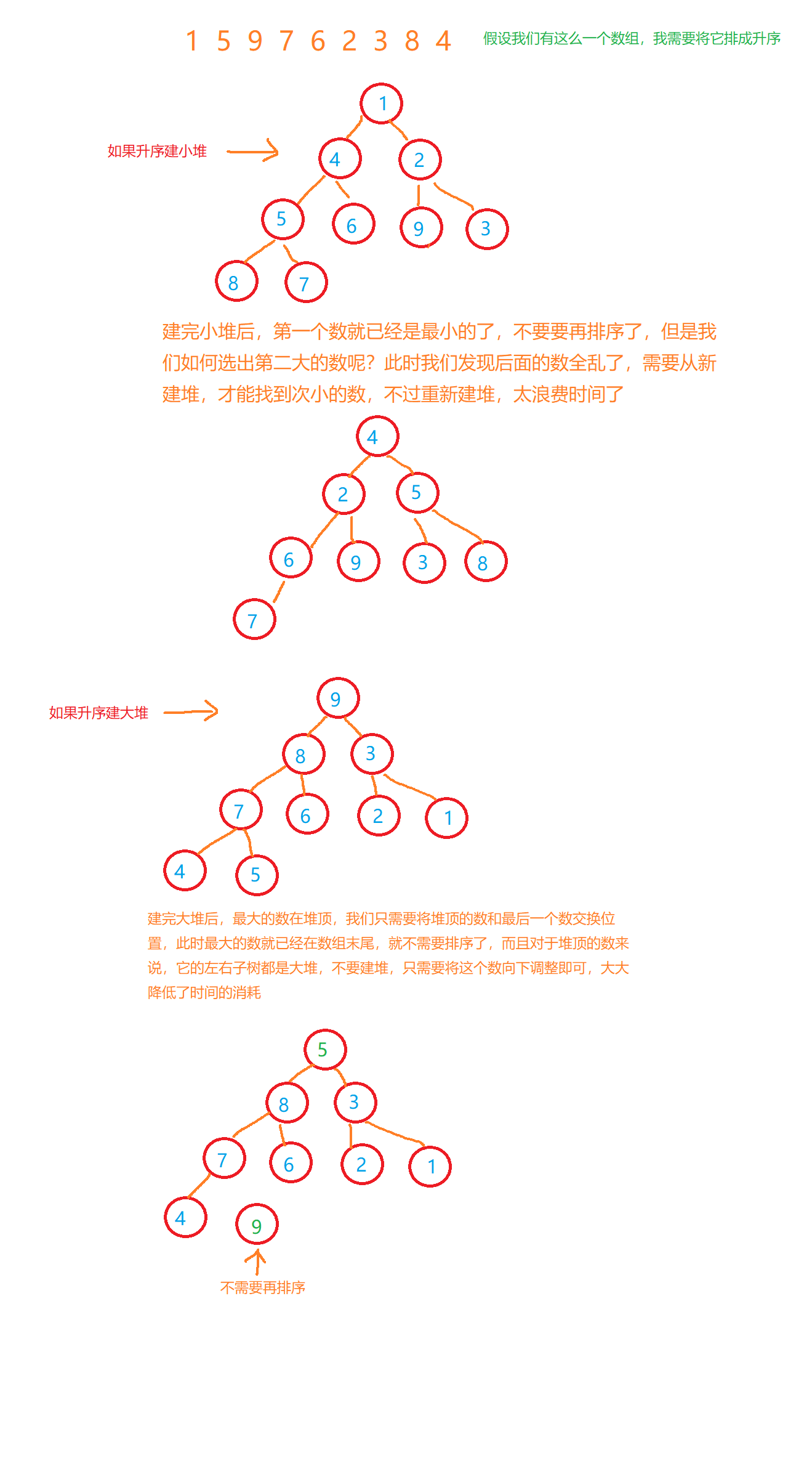2.利用堆删除思想来进行排序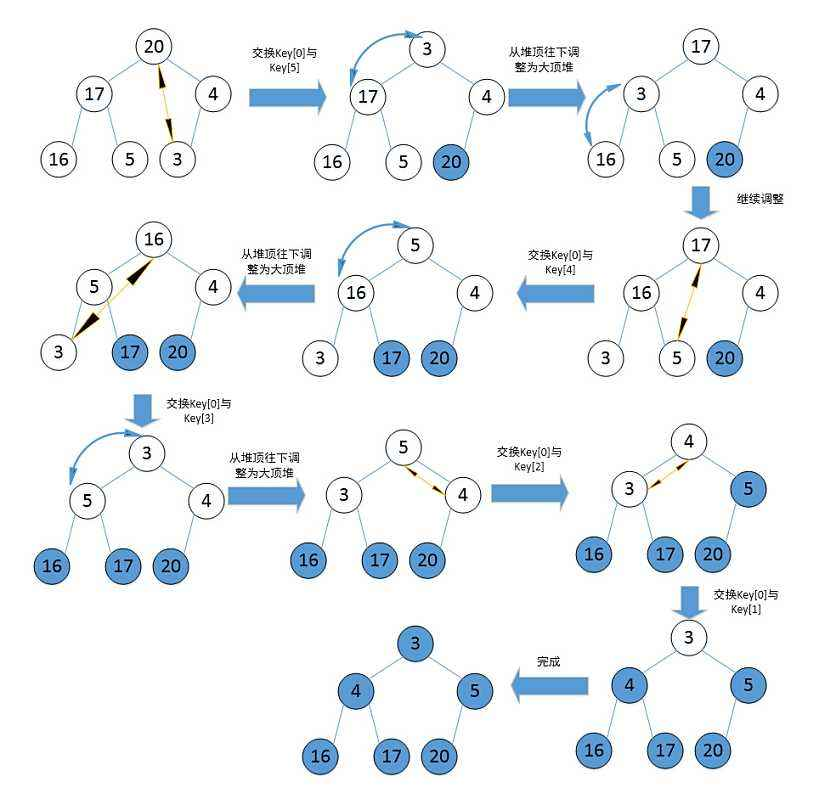``````void AdjustDown(int* a, int n, int root)//升序建大堆，降序建小堆，建堆的时间复杂度O(N)
{
int parent = root;
int child = parent * 2 + 1;//默认是左孩子
while (child < n)
{
if (child + 1 < n && a[child] < a[child + 1])
{
child += 1;
}
if (a[child] > a[parent])
{
Swap(&a[child], &a[parent]);
parent = child;
child = parent * 2 + 1;
}
else{
break;
}
}
}

void heapSort(int* a, int n)
{
for (int i = (n - 2) / 2; i >= 0; i--)
{
}

int end = n - 1;
while (end > 0)
{
Swap(&a, &a[end]);
end--;
}
}
``````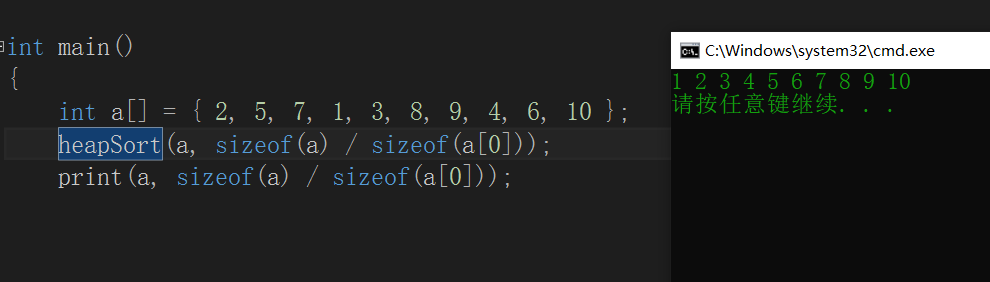## 2.TOP-K问题

TOP-K问题：即求数据结合中前K个最大的元素或者最小的元素，一般情况下数据量都比较大。

1. 用数据集合中前K个元素来建堆

• 找最大的前K个，建立K个数的小堆
• 找最小的前K个，建立K个数的大堆
2. 用剩余的N-K个元素依次与堆顶元素来比较，不满足则替换堆顶元素将剩余N-K个元素依次与堆顶元素比完之后，堆中剩余的K个元素就是所求的前K个最小或者最大的元素。

``````void PrintTopK(int* a, int n, int k)
{
int* tmp = (int*)malloc(sizeof(int)* k);
for (int i = 0; i < k; i++)
{
tmp[i] = a[i];
}
for (int i = 0; i < 10000; i++)
{
if (a[i] > tmp)
{
tmp = a[i];
}
}
for (int i = 0; i < k; i++)
{
printf("%d ", tmp[i]);
}
printf("\n");
}

void TestTopk()
{
int* a = (int*)malloc(sizeof(int)* 10000);
for (int i = 0; i < 10000; i++)
{
a[i] = rand() % 10000;
}
a = 155555;
a = 155556;
a = 155557;
a = 155558;
a = 155559;
PrintTopK(a, 10000, 5);
}
``````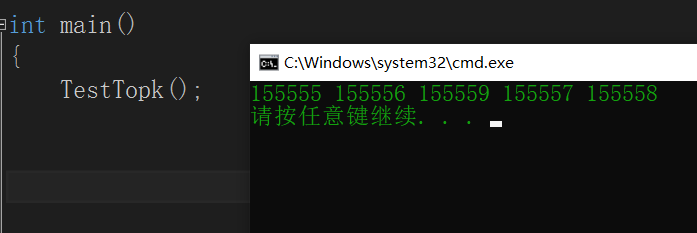# 总结